SLASEA5C March   2016  – May 2017

PRODUCTION DATA.

1. Features
2. Applications
3. Description
4. Revision History
5. Pin Configuration and Functions
6. Specifications
7. Detailed Description
1. 7.1 Overview
2. 7.2 Functional Block Diagram
3. 7.3 Audio Signal Processing Overview
4. 7.4 Feature Description
5. 7.5 Device Functional Modes
6. 7.6 Programming
1. 7.6.1 I²C Serial Control Interface
2. 7.6.2 Serial Interface Control and Timing
3. 7.6.3 26-Bit 3.23 Number Format
7. 7.7 Register Maps
8. Application and Implementation
1. 8.1 Application Information
2. 8.2 Typical Applications
1. 8.2.1 Stereo Bridge Tied Load Application
1. 8.2.1.1 Design Requirements
2. 8.2.1.2 Detailed Design Procedure
3. 8.2.1.3 Application Performance Plots
2. 8.2.2 Mono Parallel Bridge Tied Load Application
3. 8.2.3 Stereo BTL Configuration with Headphone and Line Driver Amplifier Application
4. 8.2.4 Mono Parallel Bridge-Tied Load Configuration with Headphone and Line Driver Amplifier
9. Power Supply Recommendations
10. 10Layout
1. 10.1 Layout Guidelines
2. 10.2 Layout Examples
11. 11Device and Documentation Support

• DCA|48
• DCA|48

## 6 Specifications

### 6.1 Absolute Maximum Ratings(1)

Over operating free-air temperature range (unless otherwise noted).
MIN MAX UNIT
Temperature Ambient operating temperature, TA 0 85 °C
Supply voltage DVDD, DRVDD, AVDD –0.3 4.2 V
PVDD –0.3 30 V
Input voltage DVDD referenced digital inputs –0.5 DVDD + 0.5 V
5-V tolerant digital inputs (2) –0.5 DVDD + 2.5(3) V
DR_INx DRVSS – 0.3 DRVDD + 0.3 V
Voltage at speaker output pins SPK_OUTx –0.03 32 V
Voltage at BSTRPx pins BSTRPx –0.03 39 V
Storage temperature, Tstg –40 125 °C
Stresses beyond those listed under Absolute Maximum Ratings can cause permanent damage to the device. These are stress ratings only, and functional operation of the device at these or any other conditions beyond those indicated under Recommended Operating Conditions is not implied. Exposure to absolute-maximum-rated conditions for extended periods can affect device reliability.
5-V tolerant inputs are PDN, RESET, SCLK, LRCLK, MCLK, SDIN, SDA, and SCL.
Maximum pin voltage should not exceed 6 V.

### 6.2 ESD Ratings

VALUE UNIT
V(ESD) Electrostatic discharge Human-body model (HBM), per ANSI/ESDA/JEDEC JS-001(1) ±4000 V
Charged-device model (CDM), per JEDEC specification JESD22-C101(2) ±1500
JEDEC document JEP155 states that 500-V HBM allows safe manufacturing with a standard ESD control process.
JEDEC document JEP157 states that 250-V CDM allows safe manufacturing with a standard ESD control process.

### 6.3 Recommended Operating Conditions

over operating free-air temperature range (unless otherwise noted)
MIN MAX UNIT
TA Ambient operating temperature 0 85 °C
VDD DVDD, DRVDD, and AVDD supply 2.97 3.63 V
PVDD PVDD supply 4.5 26.4(1) V
VIH Input logic high 2 V
VIL Input logic low 0.8 V
RHP Minimum HP load 16 Ω
RLD Minimum line driver load 600 Ω
RSPK(BTL) Minimum speaker load in BTL mode 4 Ω
RSPK(PBTL) Minimum speaker load in post-filter PBTL mode 2 Ω
LFILT Minimum output inductance under short-circuit condition 10 µH
For operation at PVDD levels greater than 18 V, the modulation limit must be set to 93.8% via the control port register 0x10.

### 6.4 Thermal Characteristics

THERMAL METRIC(1) DCA (48 Pins) UNIT
Special Test Case JEDEC Standard 2-Layer PCB JEDEC Standard 4-Layer PCB
RθJA Junction-to-ambient thermal resistance(1) 49.9 26.2 °C/W
RθJC(top) Junction-to-case (top) thermal resistance(2) 14.9 °C/W
RθJB Junction-to-board thermal resistance(3) 6.9 °C/W
ψJT Junction-to-top characterization parameter(4) 1.1 0.8 °C/W
ψJB Junction-to-board characterization parameter(5) 10.8 0.8 °C/W
RθJC(bottom) Junction-to-case (bottom) thermal resistance(6) 1.7 °C/W
The junction-to-case (top) thermal resistance is obtained by simulating a cold plate test on the package top. No specific JEDECstandard test exists, but a close description can be found in the ANSI SEMI standard G30-88.
The junction-to-board thermal resistance is obtained by simulating in an environment with a ring cold plate fixture to control the PCB temperature, as described in JESD51-8.
The junction-to-top characterization parameter, ψJT, estimates the junction temperature of a device in a real system and is extracted from the simulation data for obtaining RθJA, using a procedure described in JESD51-2a (sections 6 and 7).
The junction-to-board characterization parameter, ψJB, estimates the junction temperature of a device in a real system and is extracted from the simulation data for obtaining RθJA, using a procedure described in JESD51-2a (sections 6 and 7).
The junction-to-case (bottom) thermal resistance is obtained by simulating a cold plate test on the exposed (power) pad. No specific JEDEC standard test exists, but a close description can be found in the ANSI SEMI standard G30-88.

### 6.5 Electrical Characteristics

over operating free-air temperature range (unless otherwise noted)
PARAMETER TEST CONDITIONS MIN TYP MAX UNIT
VOH High-level output voltage ADR/SPK_FAULT and SDA IOH = –4 mA
DVDD = AVDD = 3 V
2.4 V
VOL Low-level output voltage IOL = 4 mA
DVDD = AVDD = 3 V
0.5 V
IIL Low-level input current Digital Inputs VI < VIL
DVDD = AVDD = 3.6 V
75 μA
IIH High-level input current VI > VIH
DVDD = AVDD = 3.6 V
75 μA
IDD 3.3-V supply current 3.3-V supply voltage
(DVDD, AVDD)
Normal mode 49 68 mA
Reset (RST = low, PDN = high) 23 38

### 6.6 Speaker Amplifier Characteristics in All Modes

over operating free-air temperature range (unless otherwise noted)
PARAMETER TEST CONDITIONS MIN TYP MAX UNIT
fSPK_AMP Speaker amplifier switching frequency 11.025-kHz, 22.05-kHz, or 44.1-kHz data rate ±2% 352.8 kHz
48-kHz, 24-kHz, 12-kHz, 8-kHz, 16-kHz, or 32-kHz data rate ±2% 384 kHz
RDS(ON) On resistance of output MOSFET (both high-side and low-side) PVDD = 15 V, TA = 25°C, die only 120
PVDD = 15 V, TA = 25°C,
160
RPD Internal pulldown resistor at output of each half-bridge making up the full bridge outputs Connected when drivers are hi-Z to provide bootstrap capacitor charge 3

### 6.7 Speaker Amplifier Characteristics in Stereo Bridge Tied Load (BTL) Mode

TA = 25°C, PVDD = 18 V, AVDD = DRVDD = DVDD = 3.3 V, audio input signal = 1-kHz sine wave, BTL, AD mode, fS = 48 kHz, RSPK = 8 Ω, AES17 filter, fPWM = 384 kHz, external components per , and in accordance with recommended operating conditions (unless otherwise specified).
PARAMETER TEST CONDITIONS MIN TYP MAX UNIT
ICN(SPK) Idle channel noise PVDD = 18 V, A-Weighted 56 µVrms
PO(SPK) Maximum continuous output power per channel PVDD = 8 V, 10% THD, 1-kHz input signal 4 W
PVDD = 13 V, 10% THD, 1-kHz input signal 10.5 W
PVDD = 18 V, 10% THD, 1-kHz input signal 20 W
SNR(SPK) Signal-to-noise ratio (referenced to 0dBFS input signal) PVDD = 18 V, A-weighted, f = 1 kHz, maximum power at THD < 1% 105 dB
THD+N(SPK) Total harmonic distortion and noise PVDD = 18 V; PO = 1 W 0.15%
PVDD = 13 V; PO = 1 W 0.13%
PVDD = 8 V; PO = 1 W 0.2%
X-Talk(SPK) Crosstalk (worst case between L-to-R and R-to-L coupling) PO = 1 W, f = 1 kHz (BD mode) –70 dB
PO = 1 W, f = 1 kHz (AD mode) –48 dB

### 6.8 Speaker Amplifier Characteristics in Stereo Post-Filter Parallel Bridge Tied Load (Post-Filter PBTL) Mode

TA = 25°C, PVDD = 18 V, AVDD = DRVDD = DVDD = 3.3 V, audio input signal = 1-kHz sine wave, BTL, AD mode, fS = 48 kHz, RSPK = 4 Ω, AES17 filter, fPWM = 384 kHz, external components per , and in accordance with recommended operating conditions (unless otherwise specified).
PARAMETER TEST CONDITIONS MIN TYP MAX UNIT
ICN(SPK) Idle channel noise PVDD = 18 V, A-Weighted 42 µVrms
PO(SPK) Maximum continuous output power per channel PVDD = 13 V, 10% THD, 1-kHz input signal 18.9 W
PVDD = 8 V, 10% THD, 1-kHz input signal 7.2 W
PVDD = 18 V, 10% THD, 1-kHz input signal 39 W
SNR(SPK) Signal-to-noise ratio (referenced to 0dBFS input signal) PVDD = 18 V, A-weighted, f = 1 kHz, maximum power at THD < 1% 105 dB
THD+N(SPK) Total harmonic distortion and noise PVDD = 18 V; PO = 1 W 0.06%
PVDD = 13 V; PO = 1 W 0.03%
PVDD = 8 V; PO = 1 W 0.15%

### 6.9 Headphone Amplifier and Line Driver Characteristics

over operating free-air temperature range (unless otherwise noted)
PARAMETER TEST CONDITIONS MIN TYP MAX UNIT
fCP Charge pump switching frequency 200 300 400 kHz
PO(HP) Headphone amplifier output power RLOAD(HP) = 32 Ω, THD+N = 1%, outputs in phase 55 mW
SNR(HP) Signal-to-noise ratio (Referenced to 55-mW output signal), RLOAD(HP) = 32 Ω, A-Weighted 101 dB
SNR(LD) Signal-to-noise ratio (Referenced to 2-Vrms output signal), RLOAD(LD) = 10 kΩ, A-Weighted 105 dB

### 6.10 Protection Circuitry Characteristics

over operating free-air temperature range (unless otherwise noted)
PARAMETER TEST CONDITIONS MIN TYP MAX UNIT
OCETHRES Overcurrent threshold for each BTL output PVDD = 15 V, TA = 25°C 4.5 A
UVETHRES(PVDD) Undervoltage error (UVE) threshold PVDD falling 4 V
UVETHRES(AVDD) Undervoltage error (UVE) threshold AVDD falling 4.1 V
UVEHYST(PVDD) UVE recovery threshold PVDD rising 4.5 V
UVEHYST(AVDD) UVE recovery threshold AVDD rising 2.7 V
OTETHRES Overtemperature error (OTE) threshold 150 °C
OTEHYST OTE recovery threshold 30 °C

### 6.11 I²C Interface Timing Requirements

MIN TYP MAX UNIT
tw(RST) Pulse duration, RST active 100 μs
td(I²C_ready) Time to enable I²C after RST goes high 13.5 ms
fSCL Frequency, SCL 400 kHz
tw(H) Pulse duration, SCL high 0.6 μs
tw(L) Pulse duration, SCL low 1.3 μs
tr Rise time, SCL and SDA 300 ns
tf Fall time, SCL and SDA 300 ns
tsu1 Setup time, SDA to SCL 100 ns
th1 Hold time, SCL to SDA 0 ns
t(buf) Bus free time between stop and start conditions 1.3 μs
tsu2 Setup time, SCL to start condition 0.6 μs
th2 Hold time, start condition to SCL 0.6 μs
tsu3 Setup time, SCL to stop condition 0.6 μs
CL Load capacitance for each bus line 400 pF

### 6.12 Serial Audio Port Timing Requirements

over recommended operating conditions (unless otherwise noted)
PARAMETER TEST CONDITIONS MIN TYP MAX UNIT
fSCLKIN Frequency, SCLK 32 × fS, 48 × fS, 64 × fS CL ≤ 30 pF 1.024 12.288 MHz
tsu1 Setup time, LRCK to SCLK rising edge 10 ns
th1 Hold time, LRCK from SCLK rising edge 10 ns
tsu2 Setup time, SDIN to SCLK rising edge 10 ns
th2 Hold time, SDIN from SCLK rising edge 10 ns
LRCK frequency 8 48 48 kHz
SCLK duty cycle 40% 50% 60%
LRCK duty cycle 40% 50% 60%
SCLK rising edges between LRCK rising edges 32 64 SCLK edges
t(edge) LRCK clock edge with respect to the falling edge of SCLK –1/4 1/4 SCLK period
tr/tf Rise/fall time for SCLK/LRCK 8 ns
LRCK allowable drift before LRCK reset 4 MCLKs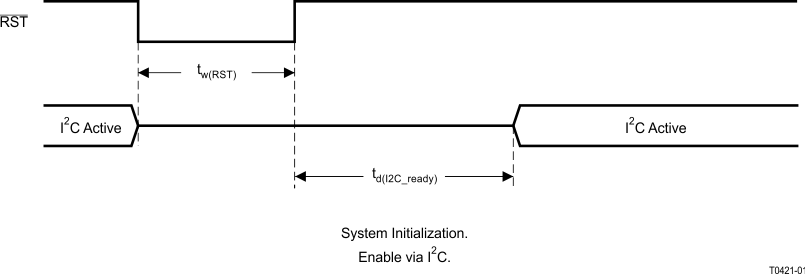NOTE:

On power up, hold the TAS5753MD RST LOW for at least 100 μs after DVDD has reached 3 V.

NOTE:

If RST is asserted LOW while PDN is LOW, then RST must continue to be held LOW for at least 100 μs after PDN is deasserted (HIGH).
Figure 1. Reset Timing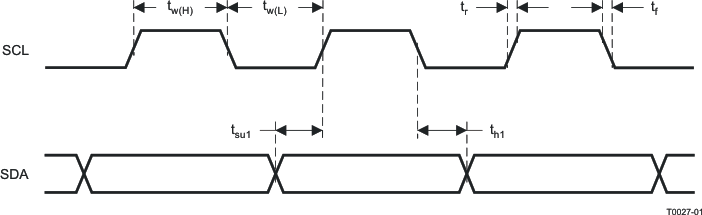Figure 2. SCL and SDA Timing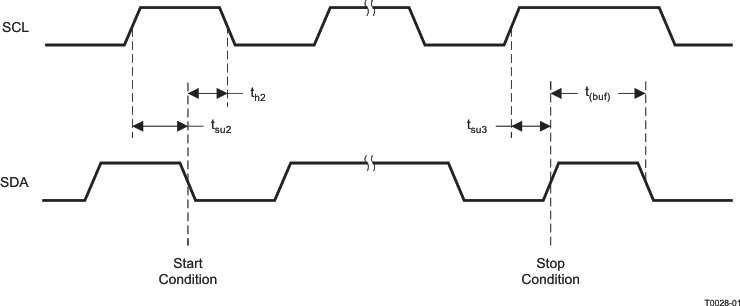Figure 3. Start and Stop Conditions Timing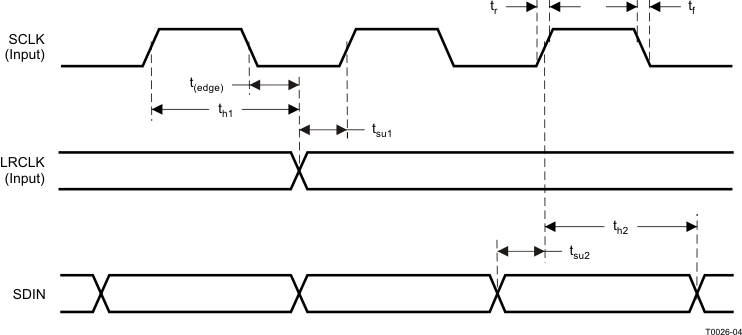Figure 4. Serial Audio Port Timing

### 6.13 Typical Electrical Power Consumption

over operating free-air temperature range (unless otherwise noted), with DVDD = 3.3 V and AVDD = PVDD, external components as specified on the EVM.
SPEAKER AMPLIFIER STATE CONFIGURATION SETTINGS VPVDD
[V]
IPVDD
[mA]
IVDD
[mA]
PDISS
(From all Supplies)
[W]
fSPK_AMP OPERATIONAL STATE
384kHz Idle RST pulled high, speaker amplifier outputs at 50/50 mute 18 20 48 0.51
Reset RST pulled low, PDN pulled high 5 21 0.16

### Table 1. Quick Reference Table

OUTPUT CONFIGURATION PLOT TITLE FIGURE NUMBER
Bridge Tied Load (BTL) Configuration Curves Output Power vs Supply Voltage – BTL Figure 5
THD+N vs Frequency – BTL Figure 6
THD+N vs Frequency – BTL Figure 7
THD+N vs Frequency – BTL Figure 8
THD+N vs Frequency – BTL Figure 9
THD+N vs Output Power – BTL Figure 10
THD+N vs Output Power – BTL Figure 11
THD+N vs Output Power – BTL Figure 12
THD+N vs Output Power – BTL Figure 13
Noise vs Supply Voltage – BTL Figure 14
Efficiency vs Output Power – BTL Figure 15
Idle Channel Current vs Supply Voltage – BTL Figure 16
Powerdown Current vs Supply Voltage – BTL Figure 17
Parallel Bridge Tied Load (PBTL) Configuration Curves Output Power vs Supply Voltage – PBTL Figure 18
Output Power vs Frequency – PBTL Figure 19
Output Power vs Frequency – PBTL Figure 20
Output Power vs Frequency – PBTL Figure 21
Output Power vs Frequency – PBTL Figure 22
THD+N vs Output Power – PBTL Figure 23
THD+N vs Output Power – PBTL Figure 24
THD+N vs Output Power – PBTL Figure 25
THD+N vs Output Power – PBTL Figure 26
Noise vs Supply Voltage – PBTL Figure 27
Efficiency vs Output Power – PBTL Figure 28
Idle Channel Draw vs Supply Voltage – PBTL Figure 29
Power Down Current vs Supply Voltage – PBTL Figure 30
Headphone Amplifier Configuration Curves Headphone Total Harmonic Distortion + Noise vs Frequency Figure 31
Headphone Total Harmonic Distortion + Noise vs Output Power Figure 32
Headphone Crosstalk vs Frequency Figure 33
Headphone Crosstalk vs Frequency Figure 34
Line Driver Configuration Curves Line Driver Total Harmonic Distortion + Noise vs Frequency Figure 35
Line Driver THD+N vs Output Voltage Figure 36
Line Driver Crosstalk vs Frequency Figure 37

#### 6.14.1 Typical Characteristics – BTL Mode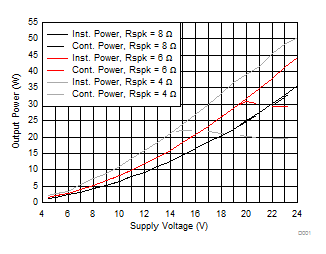THD+N = 10%
Figure 5. Output Power vs Supply Voltage – BTL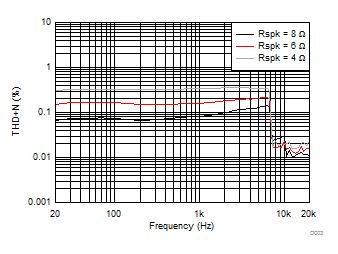PO = 1 W VPVDD = 15 V
Figure 7. THD+N vs Frequency – BTL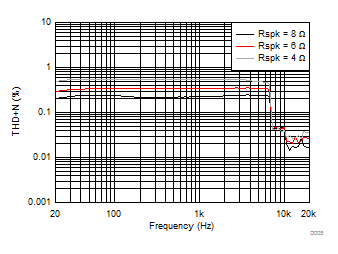PO = 1 W VPVDD = 24 V
Figure 9. THD+N vs Frequency – BTL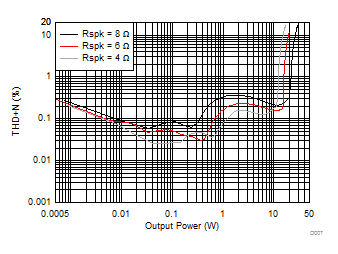Input Signal = 1 kHz VPVDD = 15 V
Figure 11. THD+N vs Output Power – BTL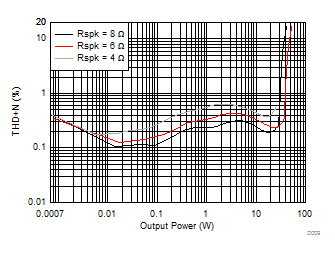Input Signal = 1 kHz VPVDD = 24 V
Figure 13. THD+N vs Output Power – BTL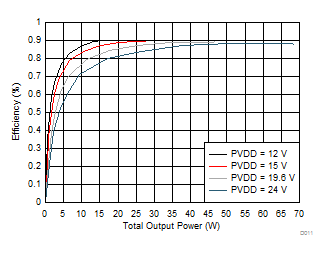RL = 8 Ω
Figure 15. Efficiency vs Output Power – BTL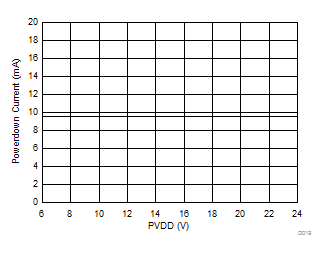LPVDD = 158 µA DVDD = 3.3 V PDN = 0
Figure 17. Powerdown Current vs Supply Voltage – BTL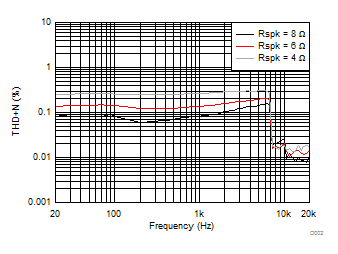PO = 1 W VPVDD = 12 V
Figure 6. THD+N vs Frequency – BTL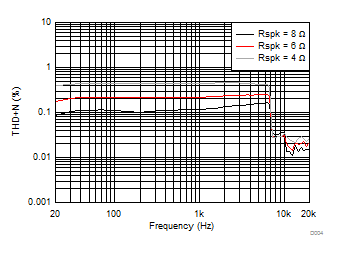PO = 1 W VPVDD = 18 V
Figure 8. THD+N vs Frequency – BTL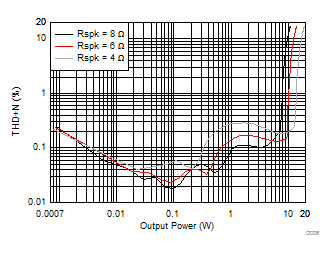f = 1 kHz VPVDD = 12 V
Figure 10. THD+N vs Output Power – BTL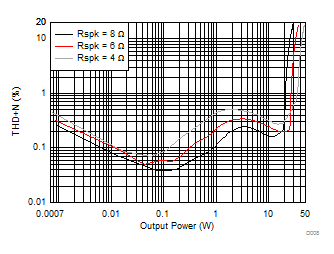Input Signal = 1 kHz VPVDD = 16 V
Figure 12. THD+N vs Output Power – BTLRL = 8 Ω
Figure 14. Idle Channel Noise vs Supply Voltage – BTL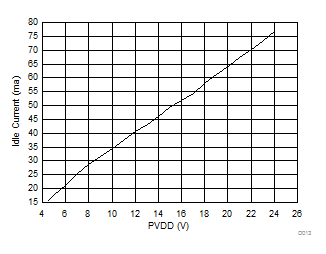RL = 8 Ω
Figure 16. Idle Channel Current vs Supply Voltage – BTL

#### 6.14.2 Typical Characteristics – PBTL Mode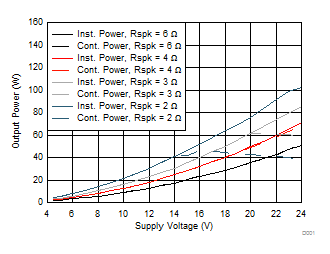THD+N = 10%
Figure 18. Output Power vs Supply Voltage – PBTL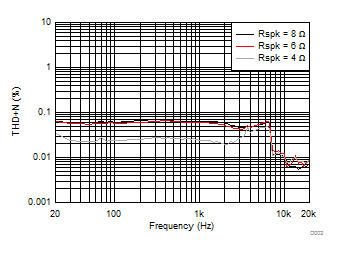PO = 1 W VPVDD = 15 V
Figure 20. THD+N vs Frequency – PBTL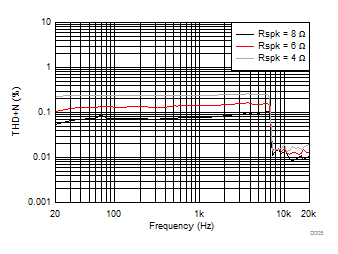PO = 1 W VPVDD = 24 V
Figure 22. THD+N vs Frequency – PBTL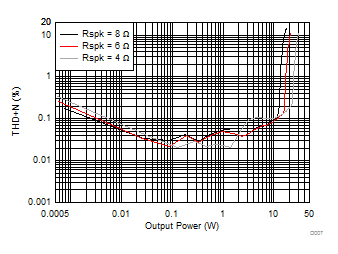f = 1 kHz VPVDD = 15 V
Figure 24. THD+N vs Output Power – PBTL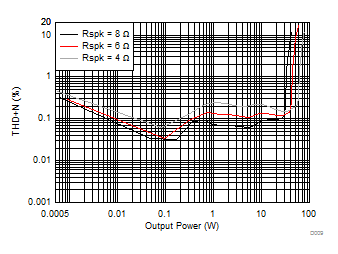f = 1 kHz Input Signal = 1 kHz Sine VPVDD = 24 V
Figure 26. THD+N vs Output Power – PBTL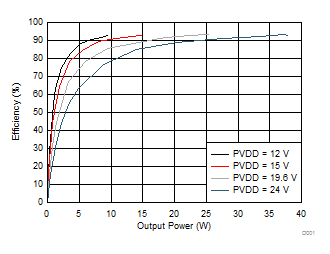RL = 8 Ω
Figure 28. Efficiency vs Output Power – PBTL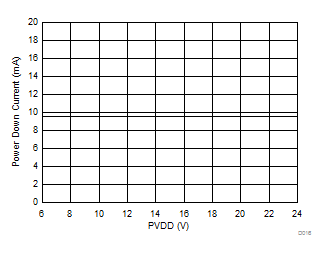IPVDD = 159 µA DVDD = 3.3 V PDN = 0
Figure 30. Power Down Current vs Supply Voltage – PBTL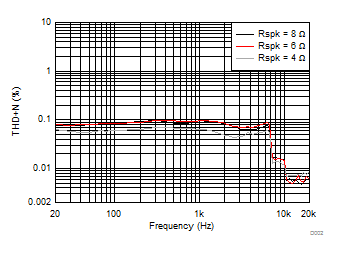PO = 1 W VPVDD = 12 V
Figure 19. THD+N vs Frequency – PBTL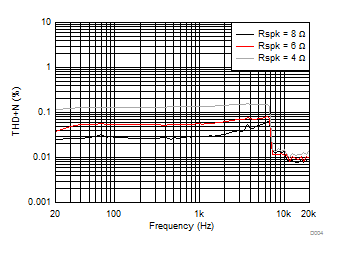PO = 1 W VPVDD = 18 V
Figure 21. THD+N vs Frequency – PBTL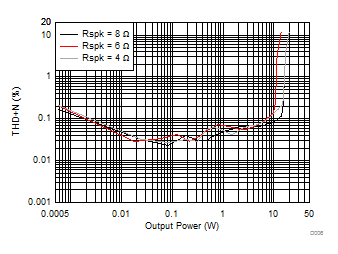f = 1 kHz VPVDD = 12 V
Figure 23. THD+N vs Output Power – PBTL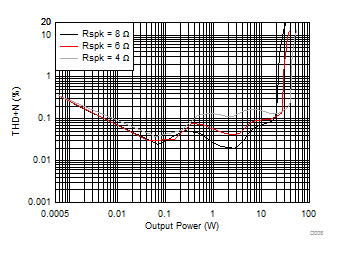f = 1 kHz Input Signal = 1 kHz Sine VPVDD = 18 V
Figure 25. THD+N vs Output Power – PBTL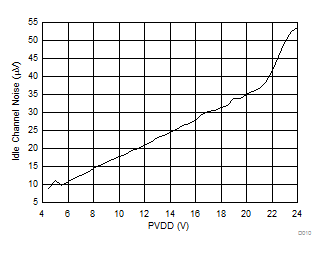RL = 8 Ω
Figure 27. Idle Channel Noise vs Supply Voltage – PBTL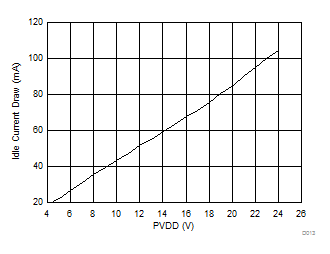RL = 8 Ω
Figure 29. Idle Channel Draw vs Supply Voltage – PBTL

#### 6.14.3 Typical Characteristics – Headphone Amplifier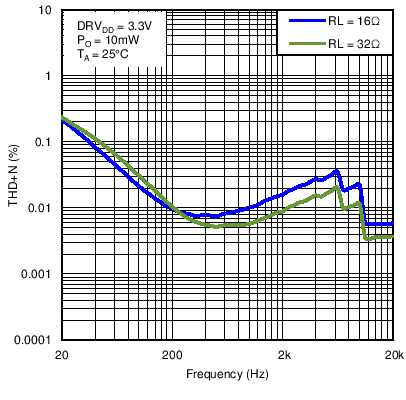DRVDD = 3.3 V PO = 10 mW TA = 25 ºC
Figure 31. Headphone Total Harmonic Distortion + Noise vs Frequency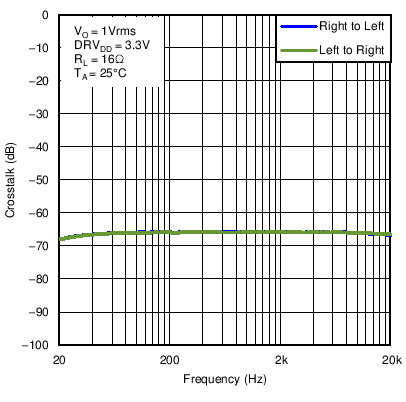DRVDD = 3.3 V VO = 1 Vrms RL = 16 Ω TA = 25 ºC
Figure 33. Headphone Crosstalk vs Frequency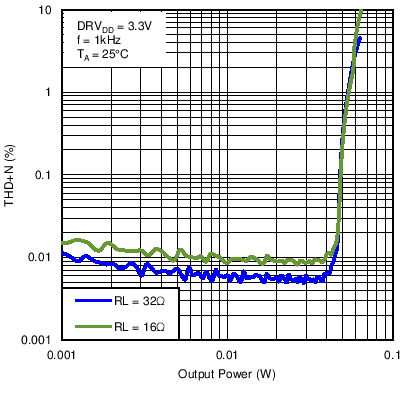DRVDD = 3.3 V f = 1 kHz TA = 25 ºC
Figure 32. Headphone Total Harmonic Distortion + Noise vs Output Power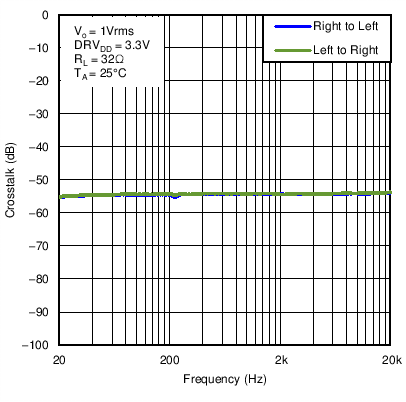DRVDD = 3.3 V VO = 1 Vrms RL = 32 Ω TA = 25 ºC
Figure 34. Headphone Crosstalk vs Frequency

#### 6.14.4 Typical Characteristics – Line Driver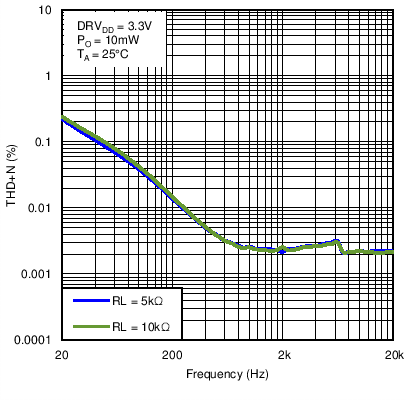DRVDD = 3.3 V PO = 10 mW TA = 25 ºC
Figure 35. Line Driver Total Harmonic Distortion + Noise vs Frequency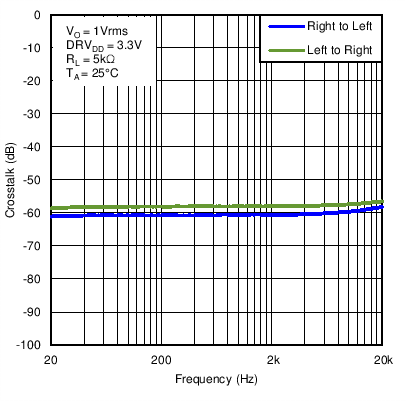DRVDD = 3.3 V VO = 1 Vrms RL = 5 kΩ TA = 25 ºC
Figure 37. Line Driver Crosstalk vs FrequencyDRVDD = 3.3 V f = 1 kHz TA = 25 ºC
Figure 36. Line Driver THD+N vs Output Voltage